The important terms and definitions used in connection with antennas and their Radiation Pattern of Antenna are

### Antenna Gain and Effective Radiated Power:

Certain types of antennas focus their Radiation Pattern of Antenna in a specific direction, as compared to an omnidirectional antenna. Another way of looking at this concentration of the radiation is to say that some antennas have gain (measured in decibels).

### Directive gain:

Directive gain is defined as the ratio of the power density in a particular direction of one antenna to the power density that would be radiated by an omnidi­rectional antenna (isotropic antenna). The power density of both types of antenna is measured at a specified distance, and a comparative ratio is established.

The gain of a Hertzian dipole with respect to an isotropic antenna = 1.5:1 power (1.5 (10 log10) = 1.76 dB).

The gain of a half-wave dipole compared to the isotropic antenna = 1.64:1 power (1.64 (10 log10) = 2.15 dB).

The wire antennas discussed in the preceding section have gains that vary from 1.64 (2.15 dB) for a half-wave dipole to 7.1 (8.51 dB) for an eight-wave dipole. These figures are for resonant antennas in free space. Similar nonresonant antennas have gains of 3.2 (5.05 dB) and 17.4 (12.4 dB) respectively. Two sets of characteristics can be obtained from the previous information:

1. The longer the antenna, the higher the directive gain.
2. Nonresonant antennas have higher directive gain than resonant antennas.

### Directivity and power gain (ERP):

Another form of gain used in connection with antennas is power gain. Power gain is a comparison of the output power of an antenna in a certain direction to that of an isotropic antenna. The gain of an antenna is a power ratio comparison between an omnidirectional and unidirectional radiator. This ratio can be expressed as: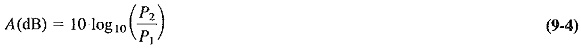where

A(dB) = antenna gain in decibels

P1 = power of unidirectional antenna

P= power of reference antenna

Another set of terms is also used in describing the performance of a transmitting system. One term is effective radiated power (erp). It applies to the field gain of the antenna and the efficiency of the transmitter.

### Radiation Measurement and Field Intensity:

The voltages induced in a receiving antenna are very small, generally in the microvolt range. Field strength measurements are thus given in microvolts per meter.

### Field intensity:

The field strength (field intensity) of an Radiation Pattern of Antenna, at a given point in space, is equal to the amount of voltage induced in a wire antenna 1 m long, located at that given point.

The field strength, or the induced voltage, is affected by a number of conditions such as the time of day, atmospheric conditions, and distance.

### Antenna Resistance:

Radiation resistance is a hypothetical value which, if replaced by an equivalent resistor, would dissipate exactly the same amount of power that the antenna would radiate.

Radiation resistance is the ratio of the power radiated by the antenna to the square of the current at the feed point.

### Antenna losses and efficiency:

In addition to the energy radiated by an antenna, power losses must be accounted for. Antenna losses can be caused by ground resistance, corona effects, imperfect dialectric near the antenna, energy loss due to eddy currents induced into nearby metallic objects, and I2R losses in the antenna itself. We can combine these losses and represent them as shown in Equation (9-5).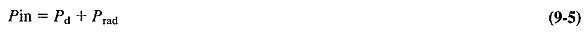where

Pin = power delivered to the feed point

Pd = power lost

Converting Equation (9-5) to I2R terms, we may state the equation as follows.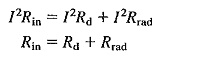From this expression we can now develop an equation for calculating antenna efficiency.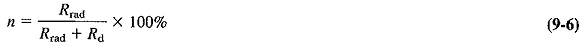Where

Rd = antenna resistance

Low- and medium-frequency antennas are least efficient because of difficulties in achieving the proper physical (resonant) length. These antennas can approach efficiencies of only 75 to 95 percent. Antennas at higher frequencies can easily achieve values approaching 100 percent. Radiation resistance values may vary from a few ohms to several hundred ohms depending on the choice of feed points and physical and electrical characteristics.

### Bandwidth, Beamwidth, and Polarization:

Bandwidth, beamwidth, and polarization are three important terms dealing respec­tively with the operating, frequency range, the degree of concentration of the Radiation Pattern of Antenna, and the space orientation of the radiated waves.

#### Bandwidth:

The term bandwidth refers to the range of frequencies the antenna will radiate effectively; i.e., the antenna will perform satisfactorily throughout this range of frequencies. When the antenna power drops to 1/2 dB), the upper and lower extremities of these frequencies have been reached and the antenna no longer performs satisfactorily.

Antennas that operate over a wide frequency range and still maintain satisfactory performance must have compensating circuits switched into the system to maintain impedance matching, thus ensuring no deterioration of the transmitted signals.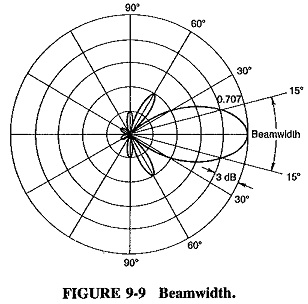#### Beamwidth:

The beamwidth of an antenna is described as the angles created by comparing the half-power points (3 dB) on the main radiation lobe to its maximum power point. In Figure 9-9, as an example, the beam angle is 30°, which is the sum of the two angles created at the points where the field strength drops to 0.707 (field strength is measured in μ/V/m) of the maximum voltage at the center of the lobe. (These points are known as the half-power points.)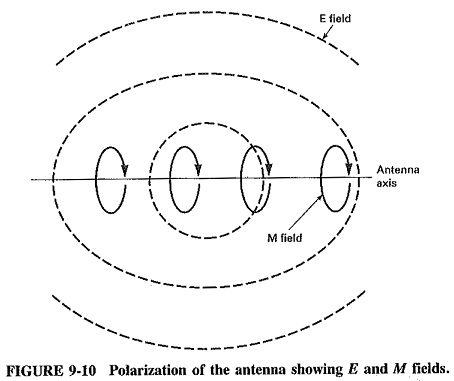#### Polarization:

Polarization of an antenna refers to the direction in space of the E field (electric vector) portion of the electromagnetic wave being radiated (Figure 9-10) by the transmitting system

Low-frequency antennas are usually vertically polarized because of ground effect (reflected waves, etc.) and physical construction methods. High-frequency antennas are generally horizontally polarized. Horizontal polarization is the more desired of the two because of its rejection to noise made by people, which is, for the most part, vertically polarized.

Scroll to Top